# Electromagnetic Induction

## Electromagnetic Induction

Electromagnetic induction occurs where there is relative motion between a conductor and a magnetic field. When this occurs an __electric current __is produced. The greater the movement, or the greater the size of the field or wire, the greater the current.

## Transformers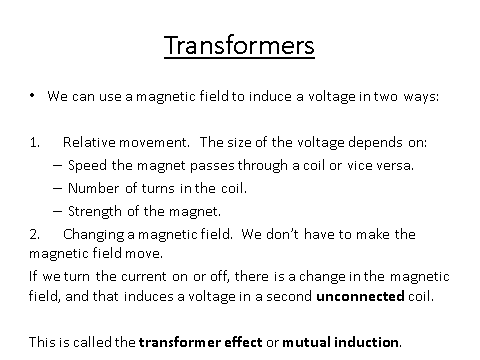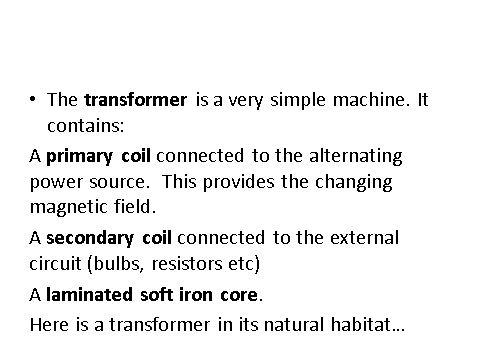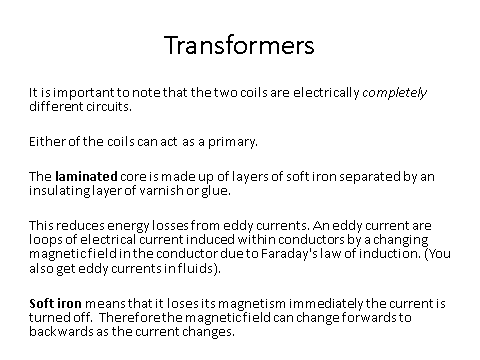## National Grid

The National Grid is a system of cables and transformers linking power stations to consumers. Electrical power is transferred from power stations to__ consumers__ using the National Grid.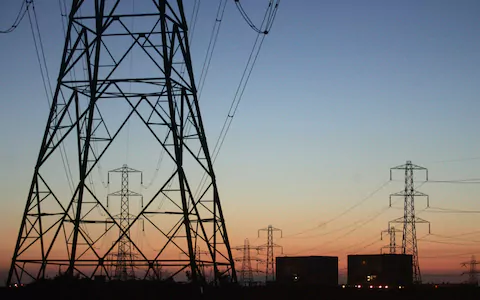In the National Grid there are two types of transformer; a step-up transformer and a step-down transformer.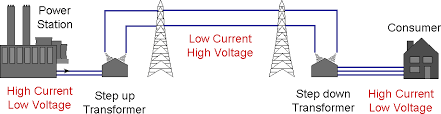• A step-up transformer increases the potential difference which causes the current to decrease.
• A step-down transformer decreases the potential difference __which causes the current to __increase.

Changing the potential difference causes a change in the current because the amount of power that is sent out and must be the same as that being received. As we remember from the previous lesson, __P = IV __so as the potential difference changes, the current must do the opposite to keep the power the same.

Although it appears like it the in the diagram above, the current in the power station and the current at the consumer are not the same.

## National Grid Transformers

The National Grid is an efficient system. This is brought about due to the transformers! (I knew you were asking why there are transformers.)

There is a step-up transformer which is at the beginning of the process which causes the current to decrease. This is important because a low current produces less resistance. Less resistance causes less heating which means less energy is wasted as heat to the environment.

So: High potential difference means low current, which means low resistance, which means less heat given off which means a more efficient system.

The step-down transformer makes the power supply a safe level __for consumers to use. The potential difference in the cables is around __132kV, this is very dangerous compared to the much safer 230V we have in the home.

## Transformer Power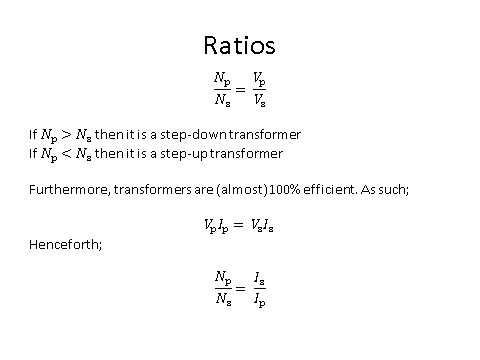﻿

What effect on current does a step-down transformer have?
increase
Why is there a step-down transformer?
Your answer should include: safe / safety
Explanation: To make the electricity supply safe for the consumer
Why is the National Grid efficient?
Your answer should include: decreases current / increases potential difference / less resistance / less heat lost
Explanation: Step up transformer increases the potential difference and decreases the current This causes the less resistance This causes less heat to be lost
What is the current in the second secondary coil of a transformer where the first potential difference is 500V, the second is 100V and the original current is 50A?
250A